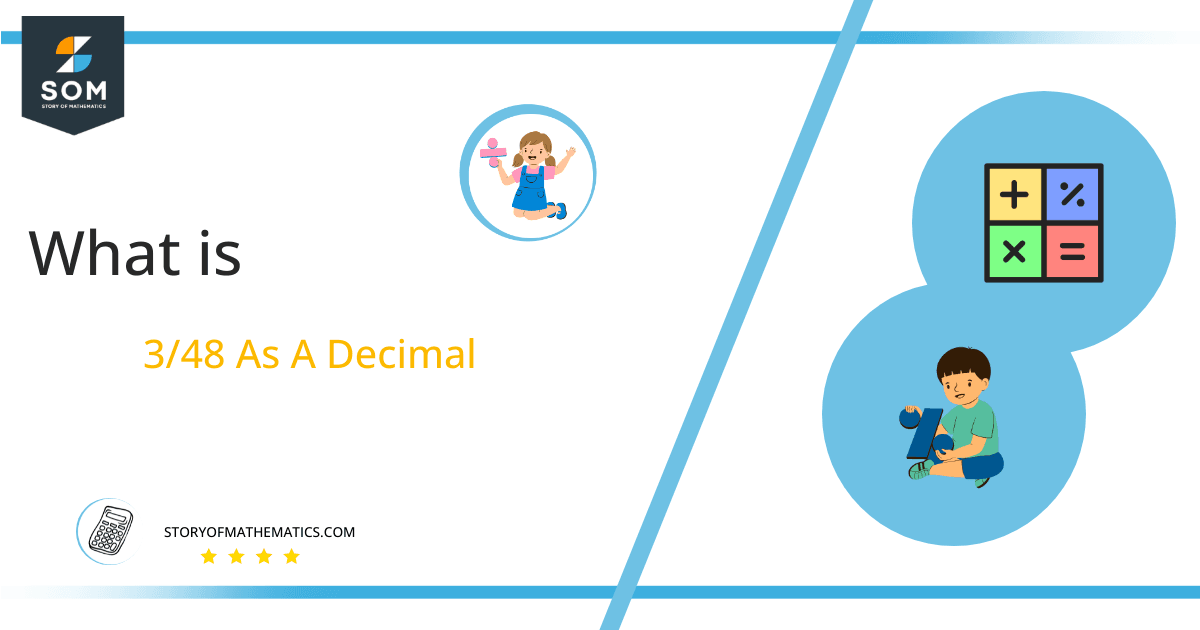# What Is 3/48 as a Decimal + Solution With Free Steps

The fraction 3/48 as a decimal is equal to 0.0625.

Proper fractions, improper fractions, and mixed fractions are the three categories under which Fractions can be categorized. Because Decimal values are more helpful in solving mathematical problems, fractions are often converted into decimal values to make them easier to understand.

Here, we are interested more in the types of division that results in a Decimal value, as this can be expressed as a Fraction. We see fractions as a way of showing two numbers having the operation of Division between them that result in a value that lies between two Integers.Now, we introduce the method used to solve said fraction to decimal conversion, called Long Division which we will discuss in detail moving forward. So, let’s go through the Solution of fraction 3/48.

## Solution

First, we convert the fraction components i.e., the numerator and the denominator, and transform them into the division constituents i.e., the Dividend and the Divisor respectively.

This can be seen done as follows:

Dividend = 3

Divisor = 48

Now, we introduce the most important quantity in our process of division, this is the Quotient. The value represents the Solution to our division, and can be expressed as having the following relationship with the Division constituents:

Quotient = Dividend $\div$ Divisor = 3 $\div$ 48

This is when we go through the Long Division solution to our problem.Figure 1

### 3/48 Long Division Method

We start solving a problem using the Long Division Method by first taking apart the division’s components and comparing them. As we have 3 and 48, we can see how 3 is Smaller than 48, and to solve this division we require that 3 be Bigger than 48.

This is done by multiplying the dividend by 10 and checking whether it is bigger than the divisor or not. If it is then we calculate the Multiple of the divisor which is closest to the dividend and subtract it from the Dividend. This produces the Remainder which we then use as the dividend later.

Now, we begin solving for our dividend 3, which after getting multiplied by 10 becomes 30.

Still, the dividend is less than the divisor, so we will multiply it by 10 again. For that, we have to add the zero in the quotient. So, by multiplying the dividend by 10 twice in the same step and by adding zero after the decimal point in the quotient, we now have a dividend of 300.

We take this 100 and divide it by 48, this can be seen done as follows:

300 $\div$ 48 $\approx$ 6

Where:

48 x 6 = 288

This will lead to the generation of a Remainder equal to 300 – 288 = 12, now this means we have to repeat the process by Converting the 12 into 120 and solving for that:

120 $\div$ 42 $\approx$ 2

Where:

48 x 2 = 96

This, therefore, produces another remainder which is equal to 120 – 96 = 24.

So, we have a Quotient generated after combining the two pieces of it as 0.062= z, with a Remainder equal to 24.Images/mathematical drawings are created with GeoGebra.